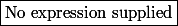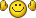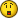# Nombres entiers positifs

alice02
Membre Naturel
Messages: 56
Enregistré le: 28 Aoû 2017, 18:28

### nombres entiers positifs

Déterminez toutes les pairesdes nombres positifs entiers tels queest un nombre entier.

pascal16
Membre Transcendant
Messages: 6060
Enregistré le: 01 Mar 2017, 14:58
Localisation: Angoulème : Ville de la BD et du FFA. gare TGV

### Re: nombres entiers positifs

(0;0) is one, maybe not the only one.

alice02
Membre Naturel
Messages: 56
Enregistré le: 28 Aoû 2017, 18:28

### Re: nombres entiers positifsno is good. Because the numbers must bechan79
Modérateur
Messages: 10324
Enregistré le: 04 Mar 2007, 21:39

### Re: nombres entiers positifs

(5;3),(1;3),(2;1),(1;2),(3;1),(2;2),(5;2),(2;5),(3;5)

alice02
Membre Naturel
Messages: 56
Enregistré le: 28 Aoû 2017, 18:28

### Re: nombres entiers positifs

Yes, but someone can post an analitic solution?
ThanksBen314
Le Ben
Messages: 20244
Enregistré le: 11 Nov 2009, 23:53

### Re: nombres entiers positifs

alice02 a écrit:no is good. Because the numbers must beBe careful : for french mathematicians a "positive" number X mean a number such that.
See for exemple the "bible" of the (french) mathematics (but not only French...), N.Bourbaki where you can read
"0 is the only number witch is both positive and negative".

And if you want to understand why there is such a difference, it's because in France, the only definition we have for a (binary) partial order is "Reflexive + Transitive + Antisymetric" sois a partiel order, butis not a partial order.
In English, there are two definitions : one for "non strict partial order" and one for "strict partial order".

And if I say to be carreful, it's because it's the same problem for other partials orders such as "to be a subset of" : When a french mathematician write, that's always mean.
Qui n'entend qu'un son n'entend qu'une sonnerie. Signé : Sonfucius

alice02
Membre Naturel
Messages: 56
Enregistré le: 28 Aoû 2017, 18:28

### Re: nombres entiers positifs

Ah ok Ben314, I didn't know it.
But someone can post a resolution?
ThanksBen314
Le Ben
Messages: 20244
Enregistré le: 11 Nov 2009, 23:53

### Re: nombres entiers positifs

Sinon, pour toutavec(sinon)
Il faut donc résoudre.
Le discriminant (en)doit être le carré d'un entier.
- On a évidementvu que.
- Mais d'un autre coté, on a la série d'équivalences suivantes (modulo que) :.
Ce qui signifie que, si, la seule possibilité pourestsauf que cette dernière équation conduit (en l'élevant au carré) àclairement sans solution (problème de parité).
Reste à traiter les cas où(c.f. EDIT çi dessous)

P.S. : Il y a surement d'autres méthodes un peu moins "rustres" (par exemple utilisant la divisibilité)...

EDIT :
- Pour y=1, l'unique solution est b=5 (et=1) qui conduit à x=2 ou bien x=3.
- Par symétrie, pour b=1, l'unique solution est y=5 qui conduit à x=2 ou bien x=3.
- Pour b=y=2 l'unique solution (double) est x=2
- Pour b=2; y=3 on a deux solutions x=1 ou bien x=5.
- Par symétrie, pour b=3; y=2 on a aussi deux solutions x=1 ou bien x=5.
Donc sauf erreur 9 solutions : (x,y){ (1;2) ; (1;3) ; (2;1) ; (2;2) ; (2;5) ; (3;1) ; (3;5) ; (5;2) ; (5;3) }
Qui n'entend qu'un son n'entend qu'une sonnerie. Signé : Sonfucius

alice02
Membre Naturel
Messages: 56
Enregistré le: 28 Aoû 2017, 18:28

### Re: nombres entiers positifs

I don't understand all passages...Can you traslate in english?
ThanksBen314
Le Ben
Messages: 20244
Enregistré le: 11 Nov 2009, 23:53

### Re: nombres entiers positifs

Lostounet, il est très fort en anglais...
Qui n'entend qu'un son n'entend qu'une sonnerie. Signé : Sonfucius

alice02
Membre Naturel
Messages: 56
Enregistré le: 28 Aoû 2017, 18:28

### Re: nombres entiers positifs

Ok.Lostounet
Messages: 9524
Enregistré le: 16 Mai 2009, 13:00

### Re: nombres entiers positifs

Lostounet, il est très fort en anglais...

Translating Ben's post:

Okay, other than that, for allwith(otherwise)

We therefore have to solve the quadratic equation.
Computing its discriminant, we get:which has to be the square of some integer.
- Obviouslysince.
- On the other hand, we have the following series of logical equivalences (granted that) :.
Which means that, if, the only possibility forishowever, squaring both sides of the last equation yieldswhich clearly has no solutions (parity incompatibility).
The only cases left to study are(look below)

P.S. : I'm sure there are other less "crude" methods (using divisibility properties of the integers for example)

EDIT :
- For y=1, the only solution is b=5 (and=1) which leads to x=2 or x=3.
- By symmetry, for b=1, the only solution is y=5 which leads us to x=2 or x=3.
- For b=y=2, the only (repeated) solution is x=2
- Take b=2; y=3 we have two solutions x=1 or x=5.
- By symmetry, for b=3; y=2 we also have two solutions x=1 or x=5.
So basically we have 9 solutions : (x,y){ (1;2) ; (1;3) ; (2;1) ; (2;2) ; (2;5) ; (3;1) ; (3;5) ; (5;2) ; (5;3) }
Merci de ne pas m'envoyer de messages privés pour répondre à des questions mathématiques ou pour supprimer votre compte.Ben314
Le Ben
Messages: 20244
Enregistré le: 11 Nov 2009, 23:53

### Re: nombres entiers positifs

Merci Lostounet....Qui n'entend qu'un son n'entend qu'une sonnerie. Signé : Sonfucius

infernaleur
Membre Irrationnel
Messages: 1001
Enregistré le: 20 Avr 2017, 19:45

### Re: nombres entiers positifs

Lostounet a écrit:
Lostounet, il est très fort en anglais...

Translating Ben's post:

Okay, other than that, for allwith(otherwise)

We therefore have to solve the quadratic equation.
Computing its discriminant, we get: (en)which has to be the square of some integer.
- Obviouslysince.
- On the other hand, we have the following series of logical equivalences (granted that) :.
Which means that, if, the only possibility forishowever, squaring both sides of the last equation yieldswhich clearly has no solutions (parity incompatibility).
The only cases left to study are(look below)

P.S. : I'm sure there are other less "crude" methods (using divisibility properties of the integers for example)

EDIT :
- For y=1, the only solution is b=5 (and=1) which leads to x=2 or x=3.
- By symmetry, for b=1, the only solution is y=5 which leads us to x=2 or x=3.
- For b=y=2, the only (repeated) solution is x=2
- Take b=2; y=3 we have two solutions x=1 or x=5.
- By symmetry, for b=3; y=2 we also have two solutions x=1 or x=5.
So basically we have 9 solutions : (x,y){ (1;2) ; (1;3) ; (2;1) ; (2;2) ; (2;5) ; (3;1) ; (3;5) ; (5;2) ; (5;3) }

This traductionalice02
Membre Naturel
Messages: 56
Enregistré le: 28 Aoû 2017, 18:28

### Re: nombres entiers positifs

Thanks Lostounet!!
I promise you that I will study french!Retourner vers ⚔ Défis et énigmes

### Qui est en ligne

Utilisateurs parcourant ce forum : Aucun utilisateur enregistré et 5 invités

Tu pars déja ?

Fais toi aider gratuitement sur Maths-forum !

Créé un compte en 1 minute et pose ta question dans le forum ;-)

Identification

Pas encore inscrit ?

Ou identifiez-vous :